# Asymptotically-efficient estimator

(diff) ← Older revision | Latest revision (diff) | Newer revision → (diff)
A concept which extends the idea of an efficient estimator to the case of large samples (cf. Efficient estimator). An asymptotically-efficient estimator has not been uniquely defined. Thus, in its classical variant it concerns the asymptotic efficiency of an estimator in a suitably restricted class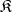of estimators. In fact, letbe a consistent estimator of a one-dimensional parameterconstructed from a random sample of size. Then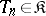if the varianceexists, and if it is bounded from below, as, by the inverse of the Fisher amount of information corresponding to one observation. An estimator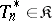which attains the lower bound just mentioned is asymptotically efficient. Under certain conditions this property is satisfied by the maximum-likelihood estimator for, which makes the classical definition meaningful. If the asymptotically-efficient estimator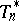exists, the magnitude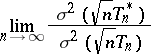is called the asymptotic relative efficiency of. Certain variants of the concept of an asymptotically-efficient estimator are due to R.A. Fisher, C.R. Rao and others.